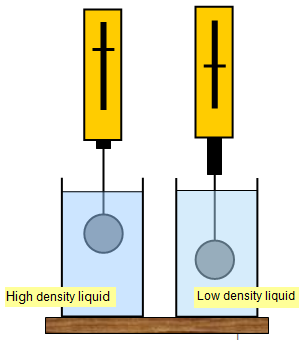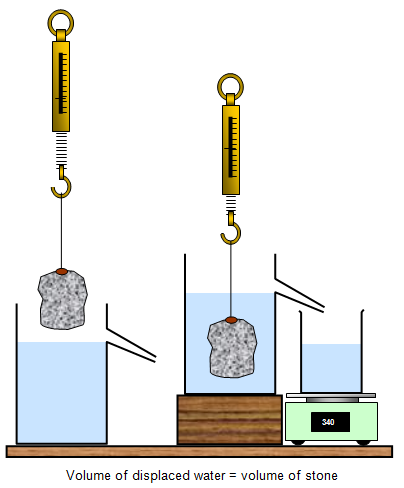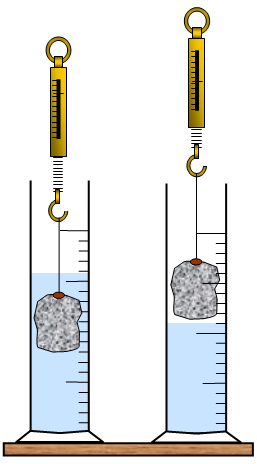# Upthrust and Archimedes principleIf you try to lift up a weight in a swimming pool and then try to lift the same weight on the edge of the pool, it feels much lighter in the water.

This was supposed to have been first explained by the Greek scientist Archimedes. He said that the water gives an upward force or upthrust on any object in it.

You can weigh an object in air and then in water and actually work out the upthrust, it is the difference between the two readings. For this reason the upthrust is often called the loss in weight of the object.

Archimedes principle states that:

When a body is partly or totally immersed in a fluid there is an upthrust that is equal to the weight of fluid displaced.

Upthrust = apparent loss of weight of object = weight in air - weight in liquid.

Example problem
A lump of iron of mass 8 kg is hung in brine of density 1100 kg/m3. If the iron has a volume of 0.001 m3, find:
(a) the density of iron;
(b) the loss of weight in brine;
(c) the apparent weight in brine.

(a) density = 8/0.001 = 8000 kg/m3
(b) loss of weight = 0.001x 1100 = 1.1x10 = 11 N
(c) apparent weight = 80 – 11 = 69 N
(Force of Earth’s gravity (g) = 10 N/kg)The weight of liquid displaced is the weight of the liquid that has been replaced by the object. The volume of this amount of liquid is equal to the volume of the object itself. The weight of fluid displaced and therefore the upthrust will be bigger if the density of the liquid is large. The upthrust in salty water (relative density = 1.1) is larger than that in water (relative density = 1.0) for the same object. This is why it is easier to swim in the sea than in a freshwater lake.## Archimedes principle and upthrustArchimedes principle says that when an object is immersed in a liquid the apparent loss of weight of an object is equal to the upthrust and this is also equal to the weight of the liquid displaced. You can prove this by the following experiments.

Weigh an object in air and then lower it into a beaker of water that is resting on a top pan balance. The reading on the spring balance will get less while the reading on the top pan balance will increase by the same amount.

If you already know the density of the liquid then you can simply measure the volume of displaced water and use mass = volume x density to find its mass. This can easily be done using a measuring cylinder as shown in the diagram.

You can see how the apparent weight of the stone gets less when it is immersed in water. If it was only partly immersed it would appear to weigh less than in air but not as little as when it is totally immersed in the water.

A set of helium balloons fixed to a thin nylon thread makes an eye-catching display in a local car showroom. It is an excellent example of upthrust in a gas. What is the equation and name for the shape of the curve? Explain your answer.A VERSION IN WORD IS AVAILABLE ON THE SCHOOLPHYSICS CD